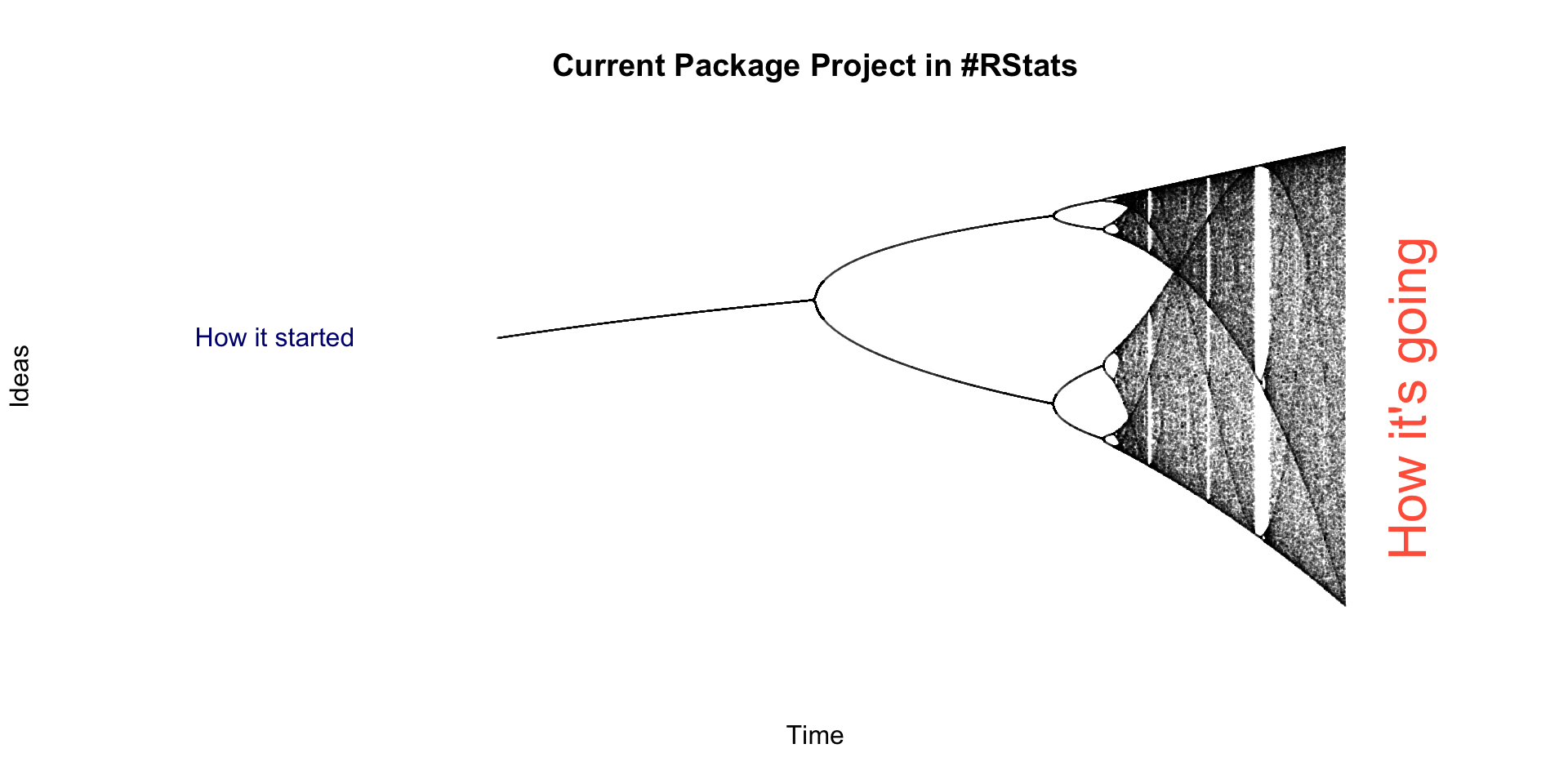# Package Development Update

``````plot(x=c(1.8, 4.2), y=c(0, 1), type = 'n', xlab = 'Time', ylab = 'Ideas', axes = F)

N <- 1000
x <- numeric(N)
x <- 0.5

for (r in seq(2.4, 4, length.out = 3000)) {
for (i in seq(N-1)) {
x[i+1] <- r * x[i] * (1 - x[i])
}

y <- unique(x[900:1000])
points(rep(r, length(y)), y, pch = '.', col = '#00000030')

}

title("Current Package Project in #RStats")
text(1.8, 0.58, pos = 4, "How it started", col = 'navy')
text(4.1, 0.1, pos = 4, srt = 90, "How it's going", col = 'tomato', cex = 2)``````